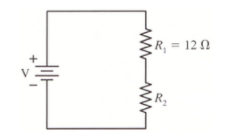# Problem: How much power is dissipated by each resistor in the figure below, in which V = 9 V and R2 = 15 Ω?

###### FREE Expert Solution

In this problem, we are dealing with power in circuits. Thus, we'll need the relation:

Power in circuits:

$\overline{){\mathbf{P}}{\mathbf{=}}{\mathbf{V}}{\mathbf{i}}{\mathbf{=}}{{\mathbf{i}}}^{{\mathbf{2}}}{\mathbf{R}}{\mathbf{=}}\frac{{\mathbf{V}}^{\mathbf{2}}}{\mathbf{R}}}$

The potential across each resistor and the current in the circuit are unknown. Thus, we'll need more relations:

Ohm's law:

$\overline{){\mathbit{i}}{\mathbf{=}}\frac{\mathbf{V}}{\mathbf{R}}}$

Lastly, we need the circuit resistance. The given resistors are in series.

Equivalent resistance:

90% (91 ratings)###### Problem Details

How much power is dissipated by each resistor in the figure below, in which V = 9 V and R2 = 15 Ω?Frequently Asked Questions

What scientific concept do you need to know in order to solve this problem?

Our tutors have indicated that to solve this problem you will need to apply the Power in Circuits concept. You can view video lessons to learn Power in Circuits. Or if you need more Power in Circuits practice, you can also practice Power in Circuits practice problems.